# KSEEB Solutions for Class 6 Maths Chapter 7 Fractions Ex 7.1

Students can Download Chapter 7 Fractions Ex 7.1 Questions and Answers, Notes Pdf, KSEEB Solutions for Class 6 Maths helps you to revise the complete Karnataka State Board Syllabus and score more marks in your examinations.

## Karnataka State Syllabus Class 6 Maths Chapter 7 Fractions Ex 7.1

Question 1.
Write the fraction representing the shaded portion.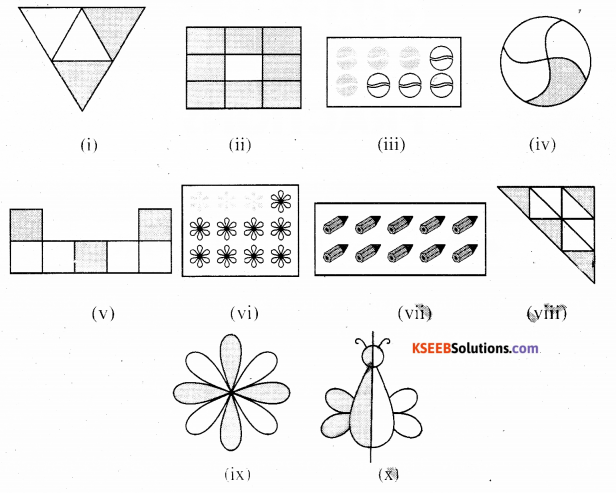i) The given figure represents 2 shaded parts out of 4 equal parts. Hence $$\frac{2}{4}$$
ii) The given figure represents 8 shaded parts out of 9 equal parts. Hence $$\frac{8}{9}$$
iii) The given figure represents 4 shaded parts out of 8 equal parts. Hence $$\frac{4}{8}$$
iv) The given figure represents 1 shaded parts out of 4 equal parts. Hence $$\frac{1}{4}$$
v) The given figure represents 3 shaded parts out of 7equalparts. Hence $$\frac{3}{7}$$
vi) The given figure represents 3 shaded parts out of 12 equal parts. Hence $$\frac{3}{12}$$
vii) The given figure represents 10 shaded parts out of 10 equal parts. Hence $$\frac{10}{10}$$
viii) The given figure represents 4 shaded parts out of 9 equal parts. Hence $$\frac{4}{9}$$
xi) The given figure represents 4 shaded parts out of 8 equal parts. Hence $$\frac{4}{8}$$
x) The given figure represents 1 shaded parts out of 2 equal parts. Hence $$\frac{1}{2}$$

Question 2.
Colour the part according to the given fraction.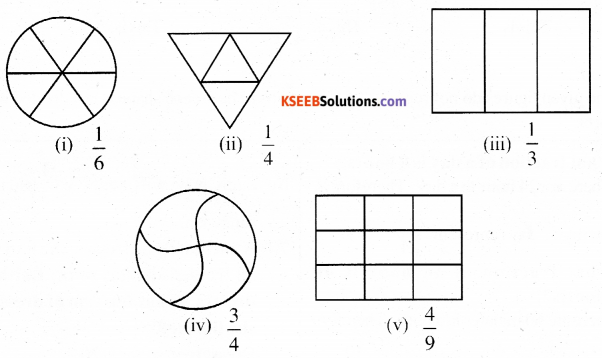Solution: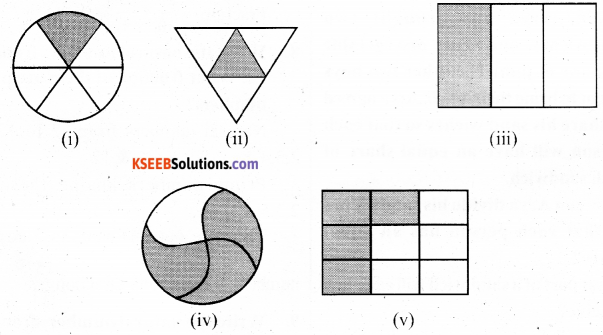Question 3.
Identify the error, if any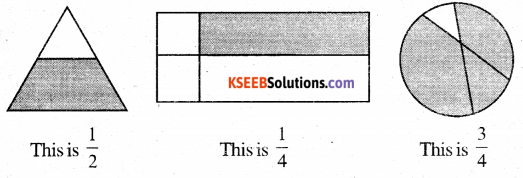Solution :
The given figures do not represent the fractions as here each shape is not divided in equal parts.

Question 4.
What fraction of a day is 8 hours?
Solution:
There are 24 hour in a day. Therefore 8, hours of a day represent $$\frac{8}{24}$$Question 5.
What fraction of an hour is 40 minutes?
Solution:
There are 60 minutes in a hour. Therefore 40, minutes of an hour represent $$\frac{40}{60}$$

Question 6.
Arya, Abhimanyu, and vivek shared lunch. Arya has brought two sandwiches, one made of vegetable and one of jam. The other two boys forgot to bring their lunch. Arya agreed to share his sand wiches so that each person will have an equal share of each sandwich.
a) How can Ary a divide his sandwiches so that each person has an equal share?
b) What part of a sand wich will each boy receive?
Solution:
a) Arya will divide each sandwich in three equal parts then,he will give one part of each sandwich to each one of them.
b) each boy will receive $$\frac{1}{3}$$ part each sandwich.

Question 7.
Kanchan dyes dresses, she had to day 30 dresses. she has so far finished 20 dresses. What fraction of dresses has she finished?
Solution:
Dress dyed so far = 20
Total dress = 30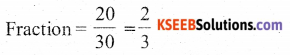Question 8.
Write the natural numbers from 2 to 12. What fraction of them are prime numbers?
Solution:
Natural numbers from 2 to 12 are 2, 3, 4, 5, 6, 7, 8, 9, 10, 11, & 12
Prime numbers among these are 2, 3, 5, & 11
Therefore, out of 11 numbers, 5 are prime numbers. It represents a fraction $$\frac{5}{11}$$

Question 9.
Write the natural numbers from 102 to 113. What fraction of them are prime numbers?
Solution:
Natural numbers from 102 to 113 are 102, 103, 104, 105, 106, 107, 108, 109, 110, 111, 112, 113.
Among these numbers, the prime numbers are 103, 107, 109 and 113
Therefore, out of 12 numbers, 4 are prime numbers, It represents a fraction $$\frac{4}{12}$$

Question 10.
What fraction of these circles have X’s in them?Solution:
There are 4 circle, out of 8, having X’s in them,
Therefore it represents a fraction $$\frac{4}{8}$$Question 11.
Kristin received a CD player for her birthday. She bought 3 CD s and received 5 others as gifts. What fraction of her total CDs did she buy and what fraction did she receive as gifts?
Solution:
Total CDs Kristin had on her birthday 3 + 5 = 8
out of 8 CDs, She bought 3 CDs and also got 5 CDs as gifts
Therefore, she bought and received CDs as gifts in a fraction of $$\frac{3}{8}$$ and $$\frac{5}{8}$$ respectively